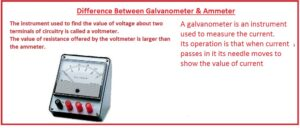Hello, friends, I hope you all are doing great. In today’s tutorial, we will discuss the Difference Between Galvanometer & Ammeter. The basic difference between galvanometer and ammeter is that the galvanometer displays the measured current direction as well magnitude but ammeter only displays the magnitude of the current. There is a moving coil in the galvanometer that moves between the permanent magnets. After the flow of current through the coil, it shows deflection. The rotation of coil and current passing through the coil is in direct relation to each other.

A galvanometer can be used as ammeter if the resistance is attached in parallel combination with the galvanometer circuit and if resistance is attached in series combination it will operate like a voltmeter. There is another name of ammeter that is ampere meter. it is due to the current unit which is ampere. To measure the value of current ammeter is connected in series combination. In today’s post, we will have a detailed look at both galvanometer and ammeter and compare them to find their differences. So let’s get started with Difference Between Galvanometer & Ammeter.

#### Difference Between Galvanometer & Ammeter

Galvanometer

• A galvanometer is an instrument used to measure the current.
• Its operation is that when current passes in it its needle moves to show the value of current
• It is considered an actuator
• It has a feature to measure the small value current that can be measured through an ammeter.
• It is a mechanical instrument.
• For operation, it needed a magnetic field.
• It is less accurate than the ammeter.
• It can measure only DC current.
• It is more sensitive than the ammeter.
• Its main parts are moving coil, suspension, and torsion head.
• Its operation and function normally based on the constant value of magnetic field.
• For constant and uniform field permanent magnet is used
• Its common typs are tangent galvanometer, mirror galvanometer, ballistic galvanometer• It used in bridge circuitry and potentiometer for current measurements.

Ammeter

• The device that measured the current passing in the circuitry is called an ammeter.
• The resistance offered by the ammeter is less than the voltmeter.
• For increase, the measuring range of the meter there should be resistance called shunt show connected to ammeter ins parallel combination.
• It normally has a feature to operates for less voltage range normally single volts or less
• The circuit for which the current is measured ammeter is attached in a series combination.
• Its accuracy is larger than the voltmeter.
• Its range can not be varied.
• It should link to supply since it has less value of resistance and can be damaged due to large current flow.
• Some meter has a range of a few mA of currents.That is detaield post about the difference between ammeter and galvanometer if you have any query ask in the comments. Thanks for reading. Have a good day.